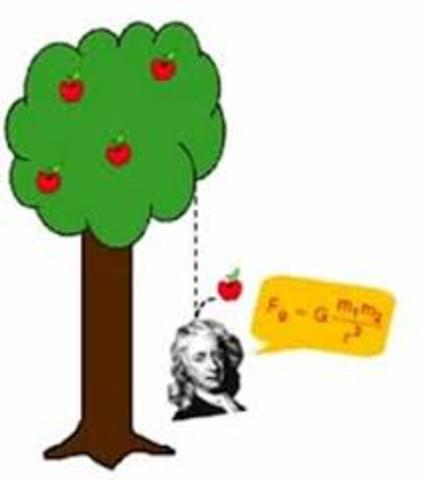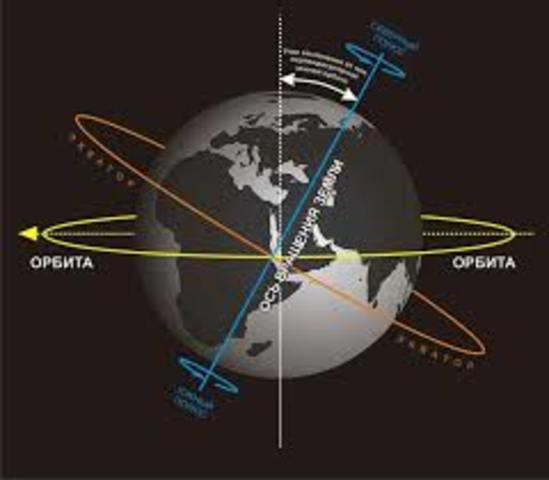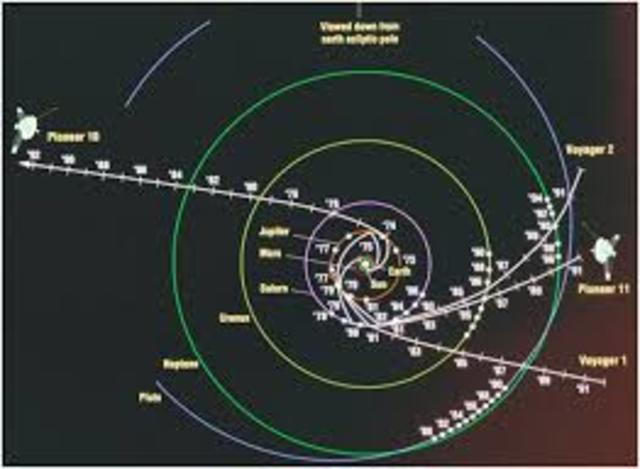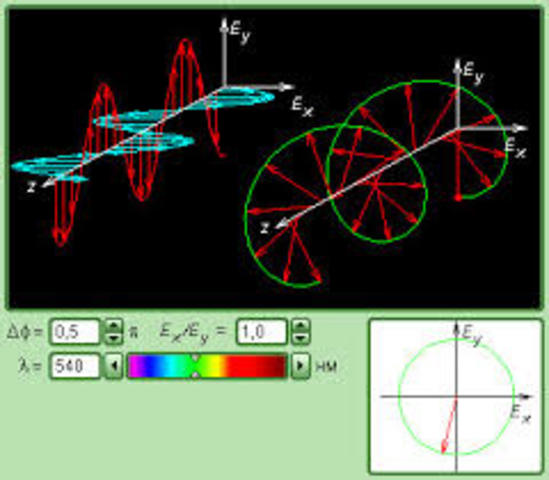• # BirthAccording to the Julian calendar, which operated in England until 1752; Or January 4, 1643 - March 31, 1727 on the Gregorian calendar) - English physicist, mathematician, mechanic and astronomer, one of the creators of classical physics
• # StudiesFinally, at the beginning of 1667 the epidemic subsided, and in April Newton returned to Cambridge. October 1, he was elected a member of Trinity College, and in 1668 he became a master.
• # First law of Ninton"Every body continues to rest in a state of rest or a uniform and rectilinear movement, until and since it does not force the forces applied to change this state."
• # Newton's second lawCause the change in the speed of bodies that are in a state of uniform motion, can change its speed only when exposed to foreign bodies.
• # Third law of NewtonBodies act on each other with forces that are the same behind the module and different in direction
• # Law of gravityGravity is the force of attraction of bodies to the Earth (to the planet). - from the law of universal gravitation. (Where M is the mass of the planet, m is the mass of the body, and R is the distance to the center of the planet). - the force of gravity from Newton's second law (where m is the mass of the body, g is the acceleration due to gravity).
• # Newton telescopeSuch a scheme of telescopes was invented by Isaac Newton in 1668. Here the main mirror directs the light onto a small flat diagonal mirror located near the focus. It, in turn, deflects a beam of light beyond the pipe, where the image is viewed through the eyepiece or photographed
• # The earth is splintered at the polesIn reality, the Earth is not an ideal ball. Because of the diurnal rotation, it is flattened from the poles; The heights of the continents are different; The shape of the surface is distorted and tidal deformations. In geodesy and astronautics, a ellipsoid of revolution or a geoid is usually used to describe the shape of the Earth.
• # Celestial MechanicsSection of astronomy, applying the laws of mechanics to study and calculate the motion of celestial bodies, primarily the solar system (the moon, planets and their satellites, comets, small bodies), and the phenomena caused by it (eclipses, etc.).
• # Differentiation and integrationIntegration can be used to find areas, volumes, central points and many useful things. But it is easiest to start with finding the area under the curve of a function like this
• # Phenomenon of light polarizationPolarization of Electromagnetic Waves For electromagnetic waves, polarization is a phenomenon of directed oscillation of the electric field strength vectors E or the magnetic field strength H.
• # Deflection of light in a gravitational fieldLight from the stars spreads in the gravitational field, which is created by other stars, the Sun, planets, etc. For the exact calculation of the gravitational deviation of the light beam, it is necessary to know the mass of the body, the distance to it from the Earth, and its coordinates on the celestial sphere
• # Interconversion of light and matterLike charged particles, the photon flux is absorbed by matter mainly due to electromagnetic interaction. However, the mechanism of this absorption is essentially different.
• # DeathIn 1725, Newton's health began to deteriorate markedly (stony disease), and he moved to Kensington not far from London, where he died at night, in a dream, on March 20 (31), 1727.